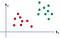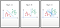# Support Vector Machine| Made Easy SVM | Machine LeaningPhoto by Neil Thomas on Unsplash

Support Vector Machine (SVM) is a supervised Machine Learning (ML) algorithm that initially developed in the 1960s and later refined in the 1990s. And it is only now, that the SVM become popular in the ML area due to its certain characteristics. SVM can be used in many ways such as —

• SVM can perform classification, regression, and even outlier detection

In this post, I will primarily discuss SVM for linear binary classification. I divide this article into three parts —

1. Intuition behind SVM

## Intuition behind SVM

We will be using 2-dimensional space for the sake of simplicity — so that we can visualize the model. In the following 2-dimensional figure — fig 1— there are some points where some are red and some are green. It is easily understandable that we can classify this into binary classification- class 1 (red) & class 2 (green) using a straight line. Fig 2- A can be a solution for linear separation and Fig 2-B can be another solution. Hence, this binary classification can be solved in many possible ways as in Fig 2-C.Fig 1Linear separation

We can draw many straight lines to separate the two classes. For each of the lines, we will achieve a similar result for the existing data points (training data)— a perfect linear separation of the two classes. Does this mean that we can use any of the straight lines? — NO

Because for unseen data (test data), based on the location of the data and the straight line — the consequences might be different meaning that the new data can either be in class one or in class 2. Hence, We must search for an optimal straight line that can generalize the model and perform well for unseen or test data. Let’s see an example — why we need to search for an optimal straight line.

We used two different lines to classify the data in Fig 1. In Fig 3-A the new data or test data is classified as a red point while in Fig 3-B, the point classified as green though the location of the test data is at the same place.

SVM searches for an optimal straight line for the binary classification using two other straight on both sides as in Fig 4. Assume that, all the red points in class +1 and all the green points in class -1. In SVM, we find the linear line at first and then start to stretch our hands on both sides until we touch the nearest point on both sides. When we find the nearest point, we draw a parallel line and call it a marginal line. In Fig 4, the red marginal line passes through one of the red points (class +1), we call it a positive marginal line and similarly, we call the green line a negative marginal line. The distance between the positive and negative marginal line is the marginal distance. In SVM, our main objective is to maximize the marginal distance to perform the classification task.

So far, we discussed all the terms in 2-dimensional space, now we generalize the terms for higher-dimensional space — Fig 5. A linear line in higher dimensional space is called a hyperplane, and a point in higher dimension is a vector. The vectors that pass through the marginal hyperplanes are called support vectors. The Support vectors play a crucial role in developing the SVM algorithm mainly deciding the location of marginal hyperplanes.

We will discuss the other two parts soon!

Ph.D. Student in Analytics and Data Science, Kennesaw State University. Research focus: Improving and understanding model performance for Imbalanced datasets.

## More from Mohammad Masum

Ph.D. Student in Analytics and Data Science, Kennesaw State University. Research focus: Improving and understanding model performance for Imbalanced datasets.